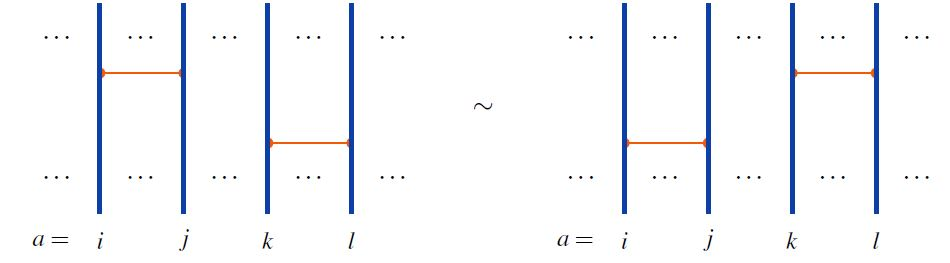# nLab 2T relation

Contents

### Context

#### Knot theory

knot theory

Examples/classes:

Types

knot invariants

Related concepts:

category: knot theory

# Contents

## Idea

In knot theory the 2-term relations on horizontal chord diagrams are the following relations in the linear span of the set of horizontal chord diagrams:

For pairwise distinct indices $i,j,k,l$, the $(i,j)$-chord generator commutes with the $(k,l)$-chord generator, for instance:graphics from Sati-Schreiber 19c

Together with the horizontal 4T relations these are the relations respected by horizontal weight systems.